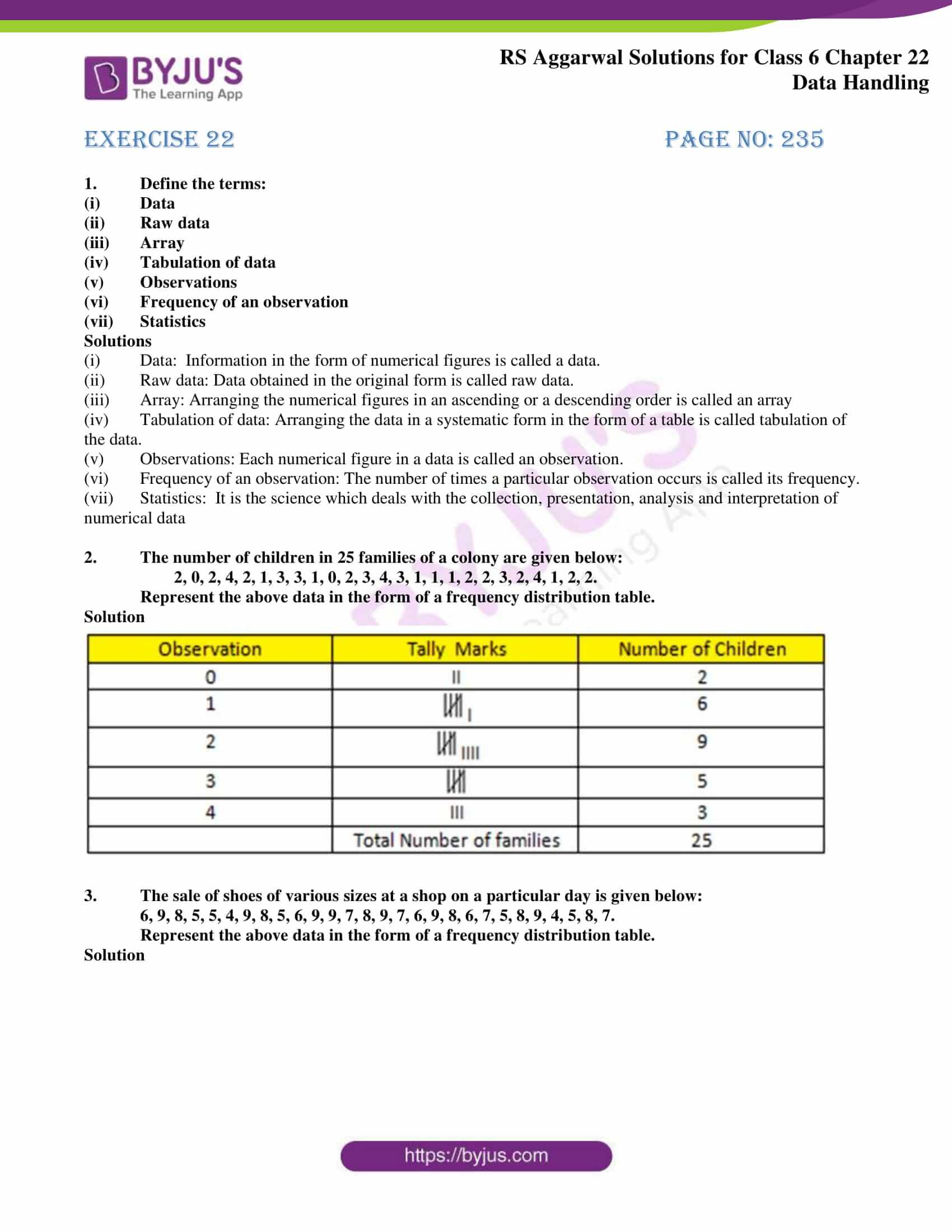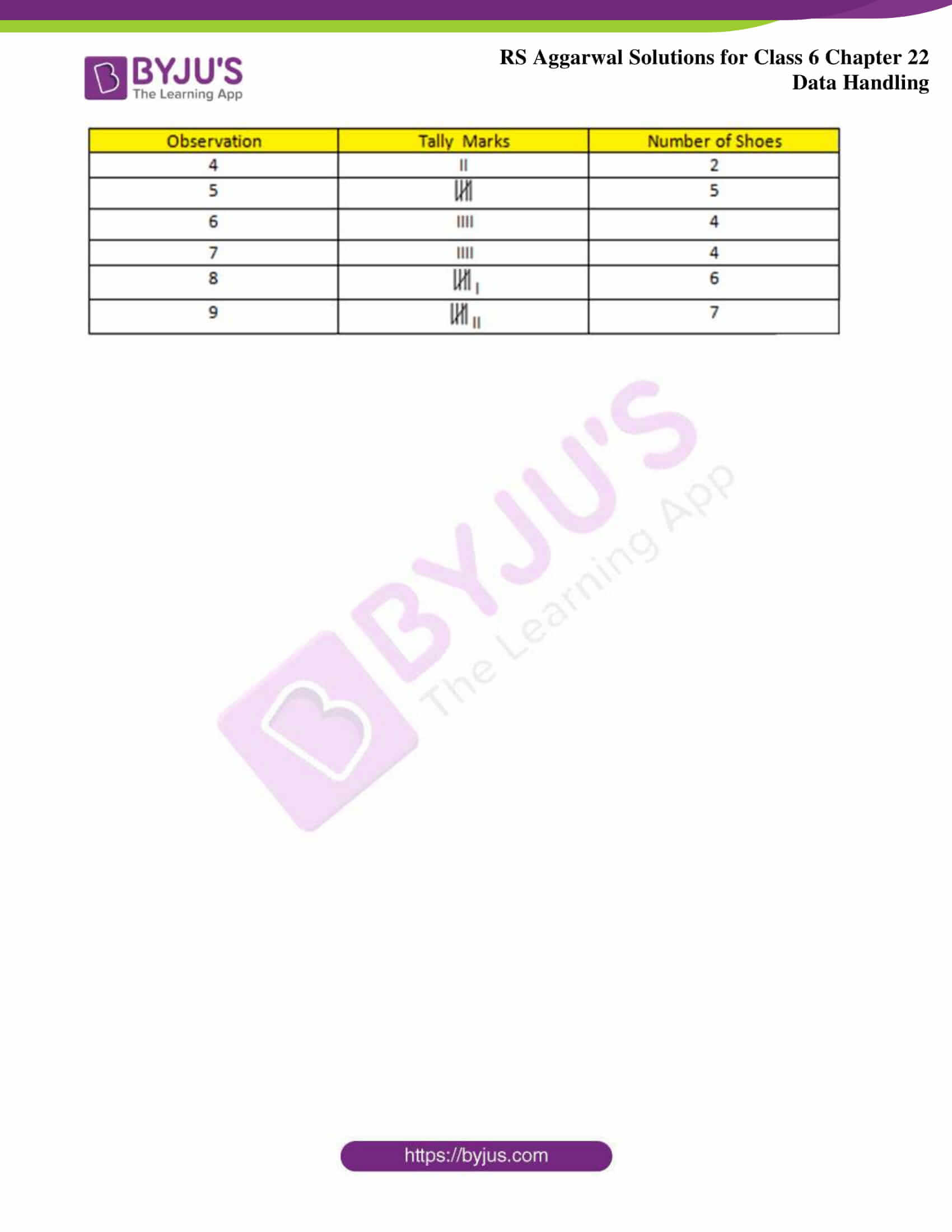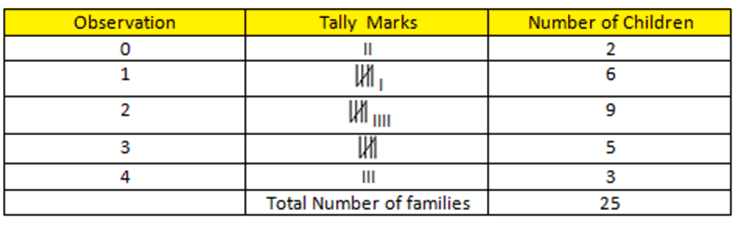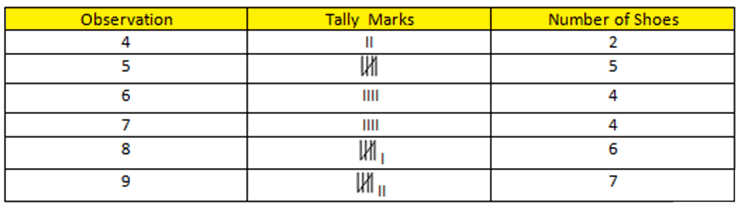# RS Aggarwal Solutions for Class 6 Maths Chapter 22 Data Handling

RS Aggarwal Solutions for Class 6 Chapter 22 Data Handling are available here. This textbook has a great collection of questions. Students have been using this textbook for Data Handling to practice important questions. Students who solve textbook questions and compare their answers with the solutions given here will score excellent marks in examination. Continuously revising these RS Aggarwal Solutions, will help them understand the chapter concept in depth.

Mathematics experts have solved RS Aggarwal textbook questions in PDF format for students of Class 6 to help them in the examination. These solutions are free to download. Download PDF of Class 6 Chapter 22 in their respective links.

## Download PDF of RS Aggarwal Solutions for Class 6 Chapter 22 – Data Handling### Access answers to chapter 22 – Data Handling

Exercise 22 PAGE NO: 235

1. Define the terms:

(i) Data

(ii) Raw data

(iii) Array

(iv) Tabulation of data

(v) Observations

(vi) Frequency of an observation

(vii) Statistics

Solutions

(i) Data: Information in the form of numerical figures is called a data.

(ii) Raw data: Data obtained in the original form is called raw data.

(iii) Array: Arranging the numerical figures in an ascending or a descending order is called an array

(iv) Tabulation of data: Arranging the data in a systematic form in the form of a table is called tabulation of the data.

(v) Observations: Each numerical figure in a data is called an observation.

(vi) Frequency of an observation: The number of times a particular observation occurs is called its frequency.

(vii) Statistics: It is the science which deals with the collection, presentation, analysis and interpretation of numerical data

2. The number of children in 25 families of a colony are given below:

2, 0, 2, 4, 2, 1, 3, 3, 1, 0, 2, 3, 4, 3, 1, 1, 1, 2, 2, 3, 2, 4, 1, 2, 2.

Represent the above data in the form of a frequency distribution table.

Solution3. The sale of shoes of various sizes at a shop on a particular day is given below:

6, 9, 8, 5, 5, 4, 9, 8, 5, 6, 9, 9, 7, 8, 9, 7, 6, 9, 8, 6, 7, 5, 8, 9, 4, 5, 8, 7.

Represent the above data in the form of a frequency distribution table.

Solution### RS Aggarwal Solutions for Class 6 Chapter 22 Data Handling

Chapter 22 consists of 1 exercise. All questions of RS Aggarwal for Class 6 Chapter 22 have been solved clearly so that students can understand them easily. Let’s have a glance at the topics included in this chapter.

• Data
• Raw data
• Array
• Tabulation of data
• Observations
• Frequency of an observation
• Statistics

After the conclusion of a research project, the process of ensuring that the research data is stored or disposed of in a safe and secure manner is known as Data Handling. Keeping the books in a systematic manner in libraries, recording water levels in rivers etc. are examples of Data Handling.